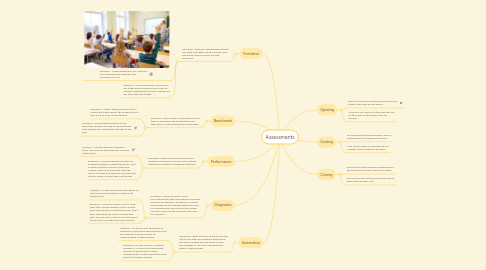# AssessmentsCaitie Huriega
Get Started. It's FreeAssessments## 1. Formative

### 1.1. Definition: These are assessments that are low stake and taken casually during class. The grade value is usually not that important.

1.1.1. Example 1: Asking students to do a thumbs up or thumbs down whether they understand or not

1.1.2. Example 2: Having students complete an exit ticket before leaving class to get an average understanding if they understood the topic that was taught

## 2. Summative

### 2.1. Definition: These are given at the end of the unit to see what the students learned and are usually graded and are used to place the students at a level of understanding based on their grades

2.1.1. Example 1: An end of unit example is an example of summative assessment to give the students a grade and get an understanding of their learning

2.1.2. Example 2:Writing a paper is another example of a summative assessment because students have to work independently to show what they know about to assigned prompt

## 3. Benchmark

### 3.1. Definition: These types of assessment are to track or see where the students are and their level of understanding and knowledge

3.1.1. Example 1: iStation testing is taken once a month and tracks where the students are in their level of ELAR understanding

3.1.2. Example 2: STAAR testing is done to see where the students are and to see what they have learned and understood throughout the year

## 4. Performance

### 4.1. Definition: These assessments are having students complete an actual task or activity rather than answering numbered questions

4.1.1. Example 1: Having students complete a Story Map over the story that was recently read to them

4.1.2. Example 2: Having students complete an experiment based of what they know, such as paper airplane contest or egg drop activity, these are conducted after the lesson is taught and students complete the activity based on what they just learned

## 5. Diagnostic

### 5.1. Definition: These are pretty much pre-assessments that the students complete and give the teacher a foundation for what the students know and their beginning level of understanding, which helps the teacher form her lesson on the level that best suits the students

5.1.1. Example 1: A short quiz before the lesson to get a quick understanding of where the students are

5.1.2. Example 2: An anchor chart such as a KWL chart that has the students come up with their own answers of what they know, and it goes along with the lesson of what they want to know and is used as an assessment at the end to see what they have learned